## How to Calculate and Solve for Degree of Polymerization | combination | Polymer & Textile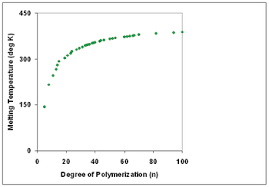The image above represents the degree of polymerization.

To compute for degree of polymerization, one essential parameter is needed and this parameter is parameter (V).

The formula for calculating the degree of polymerization:

Xn = 2V

Where:

Xn = Degree of Polymerization
V = Parameter

Let’s solve an example;
Find the degree of polymerization with a parameter of 28.

This implies that;

V = Parameter = 28

Xn = 2V
Xn = 2 (28)
Xn = 56

Therefore, the degree of polymerization is 56.

## How to Calculate and Solve for Helix Angle | Polymer & Textile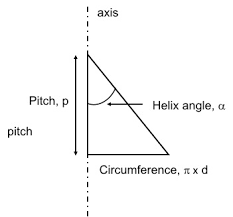The image above represents the helix angle.

To compute for helix angle, two essential parameters are needed and these parameters are screw lead (S) and barrel diameter (Db).

The formula for calculating helix angle:

θ = tan-1[S / (π x Db)]

Where;

θ = Helix Angle
Db = Barrel Diameter

Let’s solve an example;
Find the helix angle when the screw lead is 14 and the barrel diameter is 22.

This implies that;

S = Screw Lead = 14
Db = Barrel Diameter = 22

θ = tan-1[S / (π x Db)]
θ = tan-1[14/(π x 22)]
θ = tan-1[14/69.115]
θ = tan-1[0.202]
θ = 11.45°

Therefore, the helix angle is 11.45°.

## How to Calculate and Solve for Reynold’s Number | Polymer & Textile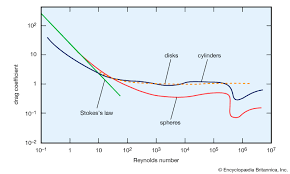The image above represents the Reynold’s number.

To compute for  Reynold’s number, four essential parameters are needed and these parameters are density of fluid (ρ), mean flow velocity (v), diameter of die (d) and viscosity (μ).

The formula for calculating Reynold’s number:

Re = ρvd / μ

Where:

Re = Reynold’s Number
ρ = Density of Fluid
v = Mean Flow Velocity
d = Diameter of Die
μ = Viscosity

Let’s solve an example;
Given that density of fluid is 14, mean flow velocity is 27, diameter of die is 18 and viscosity is 10. Find the Reynold’s number?

This implies that;

ρ = Density of Fluid = 14
v = Mean Flow Velocity = 27
d = Diameter of Die = 18
μ = Viscosity = 10

Re = ρvd / μ
Re = (14)(27)(18) / 10
Re = 6804 / 10
Re = 680.4

Therefore, the Reynold’s number is 680.4.

Calculating the Density of Fluid when the Reynold’s Number, Mean Flow Velocity, Diameter of Die and Viscosity is Given.

ρ = Re x μ / vd

Where:

ρ = Density of Fluid
Re = Reynold’s Number
v = Mean Flow Velocity
d = Diameter of Die
μ = Viscosity

Let’s solve an example;
Given that Reynold’s number is 26, mean flow velocity is 14, diameter of die is 8 and viscosity is 12. Find the density of fluid?

This implies that;

Re = Reynold’s Number = 26
v = Mean Flow Velocity = 14
d = Diameter of Die = 8
μ = Viscosity = 12

ρ = Re x μ / vd
ρ = 26 x 12 / (14)(8)
ρ = 312 / 112
ρ = 2.78

Therefore, the density of fluid is 2.78.

Calculating the Mean Flow Velocity when the Reynold’s Number, Density of Fluid, Diameter of Die and Viscosity is Given.

v = Re x μ / ρd

Where:

v = Mean Flow Velocity
Re = Reynold’s Number
ρ = Density of Fluid
d = Diameter of Die
μ = Viscosity

Let’s solve an example;
Given that density of fluid is 22, Reynold’s number is 32, diameter of die is 9 and viscosity is 6. Find the mean flow velocity?

This implies that;

Re = Reynold’s Number = 32
ρ = Density of Fluid = 22
d = Diameter of Die = 9
μ = Viscosity = 6

v = Re x μ / ρd
v = 32 x 6 / (22)(9)
v = 192 / 198
v = 0.96

Therefore, the mean flow velocity is 0.96.

## How to Calculate and Solve for Moisture Regain | Polymer & Textile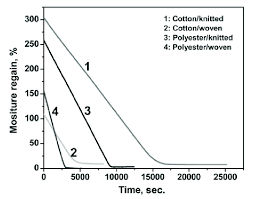The image above represents the moisture regain.

To compute for moisture regain, two essential parameters are needed and these parameters are mass of absorbed water in specimen (MW) and mass of dry specimen (MD).

The formula for calculating moisture regain:

R = MW / MD x 100

Where;

R = Moisture Regain
MW = Mass of Absorbed Water in Specimen
MD = Mass of Dry Specimen

Let’s solve an example;
Find the moisture regain when the mass of absorbed water in specimen is 13 and the mass of dry specimen is 21.

This implies that;

MW = Mass of Absorbed Water in Specimen = 13
MD = Mass of Dry Specimen = 21

R = MW / MD x 100
R = 13 / 21 x 100
R = 0.619 x 100
R = 61.90

Therefore, the moisture regain is 61.90%.

Calculating the Mass of Absorbed Water in Specimen when the Moisture Regain and the Mass of Dry Specimen is Given.

Mw = RMD / 100

Where;

MW = Mass of Absorbed Water in Specimen
R = Moisture Regain
MD = Mass of Dry Specimen

Let’s solve an example;
Find the mass of absorbed water in specimen when the moisture regain is 14 and the mass of dry specimen is 11.

This implies that;

R = Moisture Regain = 14
MD = Mass of Dry Specimen = 11

Mw = RMD / 100
Mw = 14 x 11 / 100
Mw = 154 / 100
Mw = 1.54

Therefore, the mass of absorbed water in specimen is 1.54.

## How to Calculate and Solve for Denier | Polymer & Textile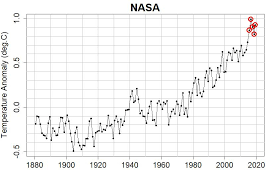The image above represents denier.

To compute for denier, two essential parameters are needed and these parameters are mass (M) and length (L).

The formula for calculating denier:

Denier = M/L x 9000

Where;

D = Denier
M = Mass
L = Length

Let’s solve an example;
Find the denier with a mass of 24 and a length of 28.

This implies that;

M = Mass = 24
L = Length = 28

Denier = M/L x 9000
Denier = 24/28 x 9000
Denier = 0.857

Therefore, the denier is 0.857.

Calculating the Mass when the Denier and the Length is Given.

M = DL / 9000

Where;

M = Mass
D = Denier
L = Length

Let’s solve an example;
Find the mass of a denier with 30 and a length of 18.

This implies that;

D = Denier = 30
L = Length = 18

M = DL / 9000
M = 30 x 18 / 9000
M = 540 / 9000
M = 0.06

Therefore, the mass is 0.06.

## How to Calculate and Solve for Burst Pressure | Polymer & Textile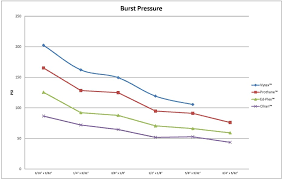The image above represents burst pressure.

To compute for burst pressure, three essential parameters are needed and these parameters are tensile strength (T), half of outside diameter of tubing (x) and half of inside diameter of tubing (y).

The formula for calculating the burst pressure:

P = T(x² – y²) / y²(1 + /y²)

Where;

P = Burst Pressure
T = Tensile Strength
x = Half of Outside Diameter of Tubing
y = Half of Inside Diameter of Tubing

Let’s solve an example;
Find the burst pressure when the tensile strength is 12, the half of outside diameter of tubing is 11 and the half of inside diameter of tubing is 7.

This implies that;

T = Tensile Strength = 12
x = Half of Outside Diameter of Tubing = 11
y = Half of Inside Diameter of Tubing = 7

P = T(x² – y²) / y²(1 + /y²)
P = 12(11² – 7²) / 7²(1 + 11²/7²)
P = 12(121 – 49) / 49(1 + 121/49)
P = 12(72) / 49(1 + 2.469)
P = 864 / 49(3.469)
P = 864 / 170
P = 5.08

Therefore, the burst pressure is 5.08.

## How to Calculate and Solve for Flexural Stress | Polymer & Textile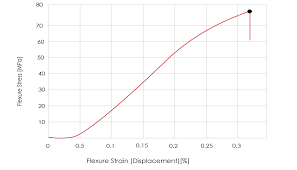The image above represents the flexural stress.

To compute for the flexural stress, three parameters are needed and these parameters are axial load at fracture point (F), width of polymer (b) and thickness of material (d).

The formula for calculating the flexural stress:

σ = F / bd

Where:

σ = Flexural Stress
F = Axial Load at Fracture Point
b = Width of Polymer
d = Thickness of Material

Let’s solve an example;
Find the flexural stress when the axial load at fracture point is 11, the width of polymer is 17 and the thickness of material is 27.

This implies that;

F = Axial Load at Fracture Point = 11
b = Width of Polymer = 17
d = Thickness of Material = 27

σ = F / bd
σ = 11 / (17)(21)
σ = 11 / 357
σ = 0.0308

Therefore, the flexural stress is 0.0308.

Calculating the Axial Load at Fracture Point when the Flexural Stress, the Width of Polymer and the Thickness of Material is Given.

F = σbd

Where:

F = Axial Load at Fracture Point
σ = Flexural Stress
b = Width of Polymer
d = Thickness of Material

Let’s solve an example;
Find the axial load at fracture point when the flexural stress is 21, the width of polymer is 10 and the thickness of material is 7.

This implies that;

σ = Flexural Stress = 21
b = Width of Polymer = 10
d = Thickness of Material = 7

F = σbd
F = 21 x 10 x 7
F = 1470

Therefore, the axial load at fracture point is 1470.

## How to Calculate and Solve for Maxwell’s Tone of Relaxation | Polymer & Textile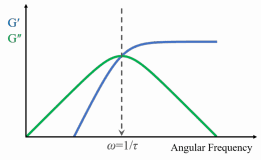The image above represents maxwell’s tone of relaxation.

To compute for the maxwell’s tone of relaxation, two essential parameters are needed and these parameters are parameter (λ) and viscosity (η).

The formula for calculating the maxwell’s tone of relaxation:

G = λη

Where:

G = Maxwell’s Tone of Relaxation
λ = Parameter
η = Viscosity

Let’s solve an example;
Given that the parameter is 15 and the viscosity is 10. Find the maxwell’s tone of relaxation?

This implies that;

λ = Parameter = 15
η = Viscosity = 10

G = λη
G = (15)(10)
G = 150

Therefore, the maxwell’s tone of relaxation is 150.

Calculating the Parameter when the Maxwell’s Tone of Relaxation and the Viscosity is Given.

λ = G / η

Where;

λ = Parameter
G = Maxwell’s Tone of Relaxation
η = Viscosity

Let’s solve an example;
Given that the maxwell’s tone of relaxation is 30 and the viscosity is 15. Find the viscosity?

This implies that;

G = Maxwell’s Tone of Relaxation = 30
η = Viscosity = 15

λ = G / η
λ = 30 / 15
λ = 2

Therefore, the parameter is 2.

## How to Calculate and Solve for Weight Average Molecular Weight | Polymer & Textile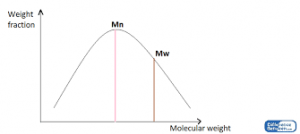The image above represents the weight average molecular weight.

To compute for the weight average molecular weight, two essential parameters are needed and these parameters are Weight Fraction (W) and Arithmetic Mean of the Molar Mass Distribution (M).

The formula for calculating the weight average molecular weight:

Mw = ΣWM

Where;

Mw = Weight Average Molecular Weight
W = Weight Fraction
M = Arithmetic Mean of the Molar Mass Distribution

Let’s solve an example;
Find the weight average molecular weight when the weight fraction is 9 and the arithmetic mean of the molar mass distribution is 18.

This implies that;

W = Weight Fraction = 9
M = Arithmetic Mean of the Molar Mass Distribution = 18

Mw = ΣWM

W M WM
9 18 162

ΣWM = 162

ΣWM = 162

Mw = 162

Therefore, the weight average molecular weight is 162.

Calculating the Weight Fraction when the Weight Average Molecular Weight and the Arithmetic Mean of the Molar Mass Distribution is Given.

W = Mw / M

Where;

W = Weight Fraction
Mw = Weight Average Molecular Weight
M = Arithmetic Mean of the Molar Mass Distribution

Let’s solve an example;
Find the weight fraction when the weight average molecular weight is 27 and the arithmetic mean of the molar mass distribution is 14.

This implies that;

Mw = Weight Average Molecular Weight = 27
M = Arithmetic Mean of the Molar Mass Distribution = 14

W = Mw / M
W = 27 / 14
W = 1.928

Therefore, the weight fraction is 1.928

## How to Calculate and Solve for Molecular Weight of Homopolymer | Polymer & Textile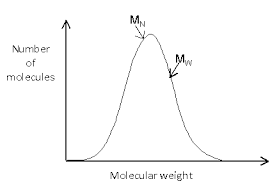The image above represents the molecular weight of homopolymer.

To compute for molecular weight of homopolymer, two essential parameters are needed and these parameters are Degree of Polymerization (x) and Sum of Masses of Repeat Unit (Mo).

The formula for calculating the molecular weight of homopolymer:

M = xMo

Where;

M = Molecular Weight of Homopolymer
x = Degree of Polymerization
Mo = Sum of Masses of Repeat Unit

Let’s solve an example;
Given that the degree of polymerization is 12 and the sum of masses of repeat unit is 24. Find the molecular weight of homopolymer?

This implies that;

x = Degree of Polymerization = 12
Mo = Sum of Masses of Repeat Unit = 24

M = xMo
M = (12)(24)
M = 288

Therefore, the molecular weight of the homopolymer is 288.

Calculating the Degree of Polymerization when the Molecular Weight of Homopolymer and the Sum of Masses of Repeat Unit is Given.

x = M / Mo

Where;

x = Degree of Polymerization
M = Molecular Weight of Homopolymer
Mo = Sum of Masses of Repeat Unit

Let’s solve an example;
Given that the molecular weight of homopolymer is 140 and the sum of masses of repeat unit is 70. Find the degree of polymerization?

This implies that;

M = Molecular Weight of Homopolymer = 140
Mo = Sum of Masses of Repeat Unit = 70

x = M / Mo
x = 140 / 70
x = 2

Therefore, the degree of polymerization is 2.